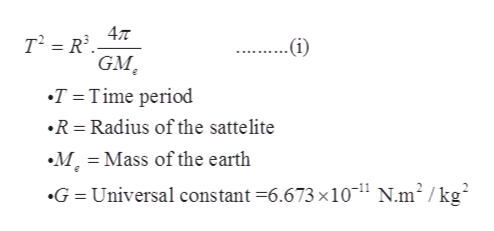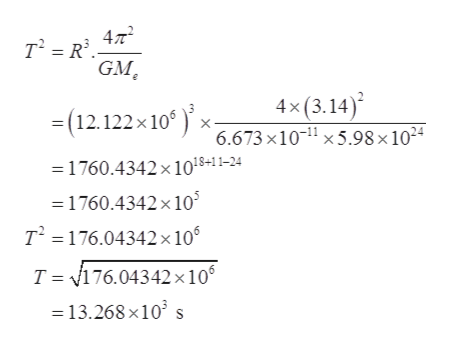# A satellite circles the earth in an orbit whose radius is 1.90 times the earth's radius. The earth's mass is 5.98 x 1024 kg, and its radius is 6.38 x 106 m. What is the period of the satellite?

Question
4 views

A satellite circles the earth in an orbit whose radius is 1.90 times the earth's radius. The earth's mass is 5.98 x 1024 kg, and its radius is 6.38 x 106 m. What is the period of the satellite?

check_circle

Step 1

Given data:

Let the radius of the satellite, R = 1.90 x (6.38 x 106 ) = 12.122 x 106 m

Mass of the earth, Me = 5.98 x 1024 kg

Step 2

For the satellite:help_outlineImage Transcriptionclose47T .(i) GM T Time period R Radius of the sattelite M Mass of the earth G = Universal constant =6.673 x1011 N.m2 / kg fullscreen
Step 3

Plugging in the given valu...help_outlineImage Transcriptionclose47 T2 R GM 4x(3.14) 6.673 x10-1 x 5.98 x 1024 =(12.122x 10 1760.4342x 1018+11-24 1760.4342 x 10 T2 176.04342x106 T 176.04342x106 13.268x10 fullscreen

### Want to see the full answer?

See Solution

#### Want to see this answer and more?

Solutions are written by subject experts who are available 24/7. Questions are typically answered within 1 hour.*

See Solution
*Response times may vary by subject and question.
Tagged in

### Other